cancel
Showing results for
Did you mean:## How to Build A Waterfall Chart to Indicate the Value Comparison Between This Year and Last Year

Table Used:

My sample table contains the data from October to December each year from 2018 to 2020.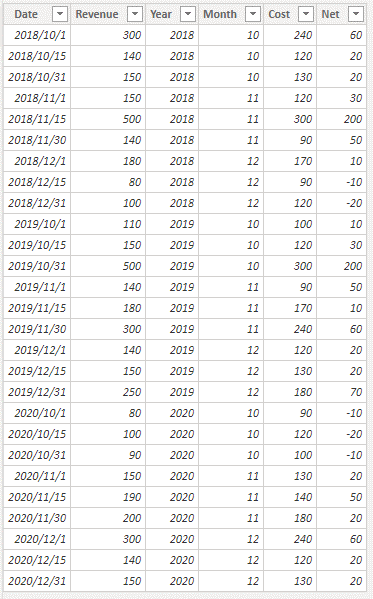Operation:

The operation steps are as follows:

1. Create a ‘Category’ Table: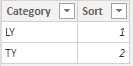2. Create a ‘Breakdown’ Table: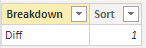3. Build a ‘Date’ Table and we can use this table to build a slicer.

`````` Date =
CALENDARAUTO(),
"Year", YEAR ( [Date] ),
"Month #", MONTH ( [Date] )
)``````

4. Build a measure as below.

``````Margin Percentage and Diff =
VAR SelectedDates =
VALUES ( 'Date'[Date] )
VAR LastYearDates =
SELECTCOLUMNS (
SelectedDates,
"LYDates",
IFERROR (
CONVERT (
( YEAR ( [Date] ) - 1 ) & "/"
& MONTH ( [Date] ) & "/"
& DAY ( [Date] ),
DATETIME
),
BLANK ()
)
),
"LY", [LYDates]
)
VAR _Currentyear =
YEAR ( TODAY () )
VAR _Currentmonth =
MONTH ( TODAY () )
VAR _NET =
IF (
ISFILTERED ( 'Date'[Date].[Year] ),
SUMX (
FILTER ( ALL ( 'Table' ), 'Table'[Date] IN SelectedDates ),
'Table'[Net]
),
SUMX (
FILTER (
ALL ( 'Table' ),
'Table'[Year] = _Currentyear
&& 'Table'[Month] = _Currentmonth
),
'Table'[Net]
)
)
VAR _Revenue =
IF (
ISFILTERED ( 'Date'[Date].[Year] ),
SUMX (
FILTER ( ALL ( 'Table' ), 'Table'[Date] IN SelectedDates ),
'Table'[Revenue]
),
SUMX (
FILTER (
ALL ( 'Table' ),
'Table'[Year] = _Currentyear
&& 'Table'[Month] = _Currentmonth
),
'Table'[Revenue]
)
)
VAR _LYNET =
IF (
ISFILTERED ( 'Date'[Date].[Year] ),
SUMX (
FILTER ( ALL ( 'Table' ), 'Table'[Date] IN LastYearDates ),
'Table'[Net]
),
SUMX (
FILTER (
ALL ( 'Table' ),
'Table'[Year] = _Currentyear - 1
&& 'Table'[Month] = _Currentmonth
),
'Table'[Net]
)
)
VAR _LYRevenue =
IF (
ISFILTERED ( 'Date'[Date].[Year] ),
SUMX (
FILTER ( ALL ( 'Table' ), 'Table'[Date] IN LastYearDates ),
'Table'[Revenue]
),
SUMX (
FILTER (
ALL ( 'Table' ),
'Table'[Year] = _Currentyear - 1
&& 'Table'[Month] = _Currentmonth
),
'Table'[Revenue]
)
)
RETURN
IF (
SELECTEDVALUE ( Category[Sort] ) = 1,
DIVIDE ( _LYNET, _LYRevenue ),
DIVIDE ( _NET, _Revenue )
)``````

5. Build a waterfall chart by Breakdown column in Breakdown Table, Category column in Category Table and the Measure.

Let's see the result.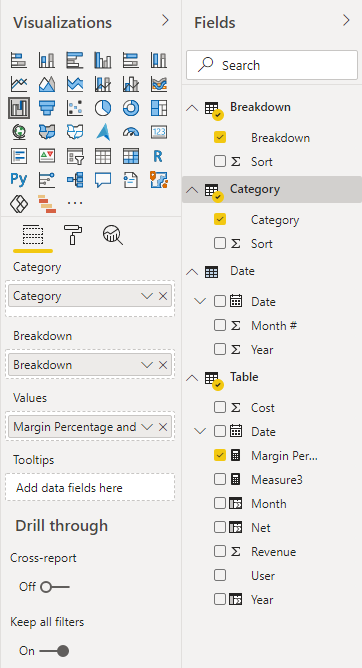Today is 2020/12/10, so the visual will compare 2020/12 with 2019/12 by default.

LY = 20.37% and TY = 16.95%. So the Diff = 16.95% -20.37% = -3.42%.When we select 2020/10 in Slicer, it will compare 2020/10 with 2019/10.

LY=31.58% and TY = -14.81%. So the Diff = -14.81% - 31.58%= -46.39%When we select 2019/10 in Slicer, it will compare 2019/10 with 2018/10.When we select 2018/10 in Slicer, it will compare 2018/10 with 2017/10.

Since there is no value for 2017 in my sample table, the value in LY will be displayed as blank.We can also select Multiple months ,quarters or years directly.

Multiple months:Compare 2020 Qtr4 with 2019 Qtr4:Due to my sample table only contains values for Otr4 from 2018 to 2020, 2020 whole year = 2020 Qtr 4 and 2019 whole year = 2019 Otr4.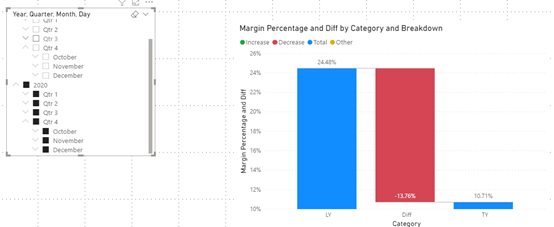See attached file for details.

Author: Rico Zhou

Reviewer: Kerry and Ula

Top Kudoed Posts
Latest Articles
Archives
Polls
What is your favorite Power BI feature release for September 2023?